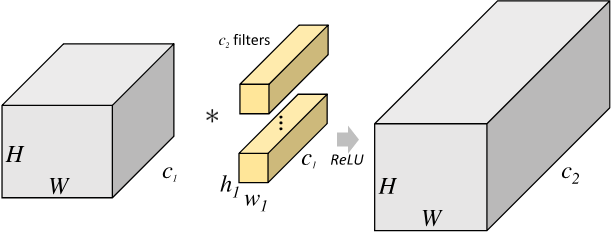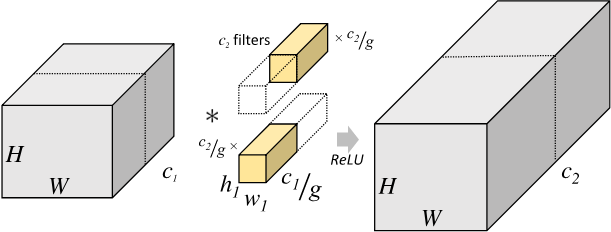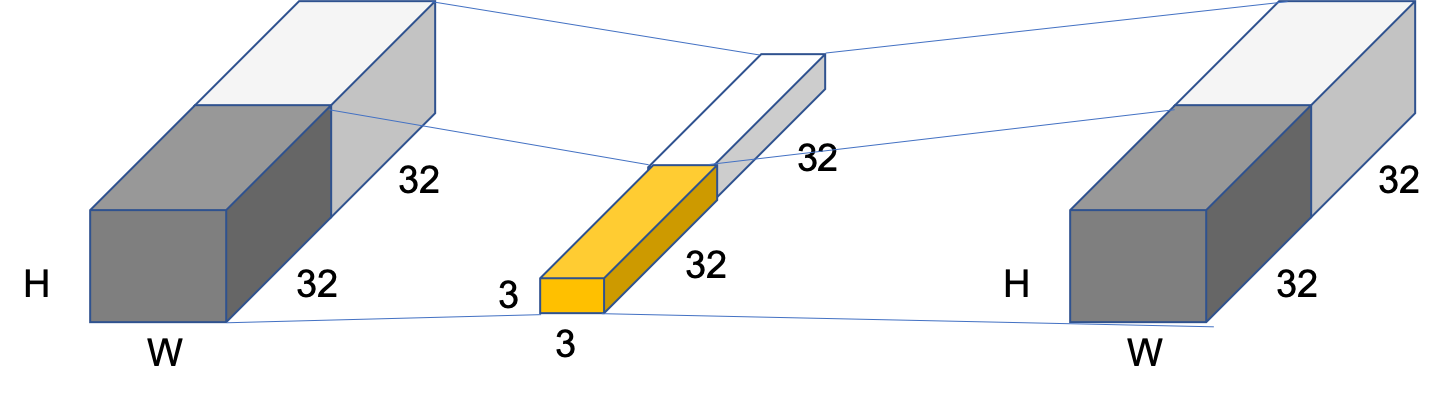# 分组卷积（Group Convolution）¶

## 二、分组卷积与标准卷积的区别¶$h_1 \times w_1 \times C_1 \times C_2$

$h_1 \times w_1 \times \frac{C_1}{g} \times \frac{C_2}{g} \times g = h_1 \times w_1 \times C_1 \times C_2 \times \frac{1}{g}$

## 三、应用示例¶